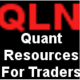# How to use statistics and probability with normal distribution for price movement analysis and exposure to reduce risk of trading loss?

(Last Updated On: September 22, 2014)Learn the Secret

Get  our 2 Free Books

Get these now which land directly to their inbox.

How to use statistics and probability with normal distribution returns for price movement analysis and exposure to reduce risk of trading loss?

There is a video below!

Can you predict which days will be up or down for trading potential? If not, see below using stats and probability as those days are not much as it appears. There are many things against you. What is below is sort of mumbo jumbo but this is a crust of my upcoming analytics signalling service. This could be scary if you fully don’t understand so see the last two paragraphs.

Sometimes, your bell curve may show fat tails which could spell out extreme profit potential or high risk potential:

Fat Tail, Risk Budgeting, Factor Analysis & Stress Testing

http://www.risk.net/risk-magazine/analysis/1603830/capturing-fat-tails

In Excel, you could use Data Analysis add in with Descriptive Statistics for summary stats. Use returns for input.

Mean is average return of index (i.e. S&P 500) over historical period of asset your analyze. It should be always be positive if you analyze S&P since 1960s.

Median is middle of data set. If median is higher than mean that says the average is more positive over the mean or opposite would be more extreme if negative.

Standard deviation (SD) is dispersion of the distribution data. i.e. 68% of standard deviation 1 lies within the mean. Could also calculate normal vs actual with percentage for each. 68% of trading days that lie within SD 1the mean of the trading distribution. 95% will be for SD 2 and 99% of SD 3.

Kurtosis and skewness means if data is normally distributed. Kurtosis is a measure of how the data has peaked. High skewness can show the data could peak more than usual within a normal distribution with fatter tails. If you have a negative skewness, that means the fat tail are negative which means than the positive tail. Extreme negative movements are more likely to happen than positive.

Probabilities for new bins of frequencies within the distribution table. Thus the frequency of ranges from within the distribution table

=# of trading days in interval/total number of trading days in distribution table (in formula cell, press F4 for \$ around on denominator to fix cell). This is represented in a cumulative representation.

You could also filter returns to understand subsets of data. We could look at an average of a subset.

Data->Filter

Average return is calculated is the same as mean.

Want to understand the negative and positive of the returns of each average return with Data->Filter

If you calculate the frequency of positive vs negative, you could estimate market direction with probability. Predict average return when S&P 500 will go up when you long for x days, you forecast the daily return of this period. ****You will soon see that the percentage the market goes up is slim with negative days, transactions costs, and volatility against you. The forecasted’ “Average return” tells you this.

Also calculate the standard deviation (SD) returns as well with SD of 1-3 above the mean. Calculate the upper and lower of each SD above/below the mean. You could find the average number of days that lie within these ranges.

In summary, be aware:

In essence, since S&P 500 moves less at the extreme, it is harder to generate revenue with other assets. In OTW, our trading opportunities are minimal when you factor in AVERAGE returns on a daily basis when volatility is low.

*** You can also say that there are greater risks in the extremity of the tails of the distribution. They can be more probable than you think so there could be days where you could get wiped out in your account. There could be for example of 1 day per 100 where the market moves more than 3% which could wipe you out if you have the position the wrong way.

You could apply this logic over any period on any asset.

Join my FREE newsletter to find out when this new signalling process gets built to protect your trading capital